Reach Us+44-1522-440391
Joint Generation of Binary and Nonnormal Continuous Data | OMICS International
Journal of Biometrics & Biostatistics

Like us on:

All submissions of the EM system will be redirected to Online Manuscript Submission System. Authors are requested to submit articles directly to Online Manuscript Submission System of respective journal.

# Joint Generation of Binary and Nonnormal Continuous Data

Hakan Demirtas*

Associate Professor of Biostatistics, Division of Epidemiology and Biostatistics (MC923), University of Illinois at Chicago, 1603 W. Taylor St., Chicago, IL, 60612, USA

*Corresponding Author:
Hakan Demirtas
Associate Professor of Biostatistics
Division of Epidemiology and Biostatistics (MC923)
University of Illinois at Chicago, 1603 W. Taylor St.
Chicago, IL, 60612, USA
Tel: 312-996-9841
E-mail: [email protected]

Received date: May 24, 2014; Accepted date: June 26, 2014; Published date: June 30, 2014

Citation: Demirtas H (2014) Joint Generation of Binary and Nonnormal Continuous Data. J Biom Biostat S12:001. doi: 10.4172/2155-6180.S12-001

Copyright: © 2014 Demirtas H. This is an open-access article distributed under the terms of the Creative Commons Attribution License, which permits unrestricted use, distribution, and reproduction in any medium, provided the original author and source are are credited.

Visit for more related articles at Journal of Biometrics & Biostatistics

#### Abstract

The use of joint models that are capable of handling different data types is becoming increasingly popular in biomedical practice. Evaluation of various statistical techniques that have been developed for mixed data in simulated environments requires concurrent generation of multiple variables. In this article, I comprehensively evaluate the unified framework proposed by Demirtas et al. for simultaneously generating binary and nonnormal continuous data given the marginal characteristics and correlation structure. I conduct this assessment in three simulated settings with synthetic bivariate and multivariate data as well as real depression score data from psychiatric research. Considering close agreement between the specified and empirically computed quantities on average, as measured by some key bias- and precision-related quantities, the methodology appears to have prospects to address the need of generating intensive data that have binary and continuous parts for simulation purposes.

#### Keywords

Tetrachoric correlation; Phi coefficient; Biserial correlation; Simulation; Random number generation; Fleishman polynomials

#### Introduction and Motivation

Most data sets include different types of variables. For instance in clustered or longitudinal designs, multiple variables are often measured or observed for each individual or at each occasion. This work is concerned with the evaluation of the framework proposed by Demirtas et al.  for simultaneously simulating binary and possibly nonnormal continuous data given the marginal characteristics of each variable as well as the linear association structure among variables in the system. The mechanism is built upon a combination of a few random variate generation routines that involve simulation of correlated binary data, multivariate normal data, a mix of binary and normal data, and multivariate nonnormal continuous data. Multivariate normal data generation is well-studied; and correlated binary data generation routine we utilize is predicated on computing tetrachoric correlations via solving a series of double integration equations assuming underlying normality before dichotomization at thresholds that correspond to the specified marginal proportions . A computational routine for joint generation of a binary and normal data, proposed by Demirtas and Doganay , constitutes an intermediate stage before augmenting the continuous part so nonnormal data can be accommodated; and is driven by the relationship among phi coefficient, point-biserial and tetrachoric (biserial) correlations. Nonnormality is handled by an extension of Fleishman’s  power polynomials procedure of expressing any given variable by the sum of linear combinations of powers of a standard univariate normal variate to the multivariate case by finding intermediate correlations that reflect the correlation structure of multivariate normal data whose components yield the nonnormal data through the coefficients of powers of normal variates [4-6]. Once such data are simulated, variables in the subsequent analyses can be treated as predictors or outcomes.

The method assumes that all variables before any transformation jointly follow a multivariate normal density. Some components are designed to be dichotomized to obtain binary variables, and some components form a basis for generating continuous data with the intended distributional features. In this random number generation (RNG) system, the proportion parameters for binary data, symmetry and elongation parameters for continuous data (as measured by the third and fourth moments) and a linear association structure among all variables need to be specified.

The following relationships among correlations should be established: 1) for the binary-binary pairs, correlations before and after dichotomization (former is computed, latter is specified); 2) for the continuous-continuous pairs, correlations before and after power polynomial transformation (former is specified, latter is computed); 3) for the binary-continuous pairs, correlations before and after dichotomizing one of the variables (former is computed, latter is specified). Unknown quantities are tetrachoric correlations, intermediate correlations and biserial correlations for Items 1, 2 and 3 above, respectively. The first one is computed through integration, the second one is involved with fairly rudimentary algebra, and the third one comes from a simple formula from the dichotomization literature. Once these operations are performed, one can form an overall correlation matrix for a multivariate normal distribution that would lead to the specified correlations after dichotomizing some of the variables via thresholds that are determined by marginal proportions for the binary part; and after applying the power transformation procedure for the continuous part. More detailed prescription is given in algorithm section. As long as some conditions outlined in algorithm hold, this method is capable of generating data that follow the specified linear association structure for all variables, means of the binary variables, and skewness and kurtosis behavior for continuous variables.

The organization of the rest of the article is as follows. In MVB data generation, I provide back- ground information on how to generate multivariate normal data, multivariate binary data through dichotomization of an underlying bivariate normal distribution whose correlation is computed by solving a numerical integration problem. Repeating this process for each possible pair gives us the overall correlation matrix. The dichotomized versions are obtained by the specified marginal proportions. I also discuss how the magnitude of the correlation changes when only one variable is dichotomized for bivariate data. In addition, the technique of power polynomials for generating multivariate continuous data is delineated in detail. In Algorithm, I outline the methodology proposed by Demirtas et al.  to generate mixed data. In stimulations, I present three simulation studies that encompass a broad range of distributional setups for bivariate and multivariate cases for evaluating the performance of this RNG technique by commonly accepted accuracy and precision measures. Two of these simulated scenarios use synthetic data, and one is devised around a real depression score data from psychiatric research. Discussion includes discussion, concluding remarks and future directions.

#### Getting Ready: Fundamentals

In this section, I give key characteristics of multivariate normal (MVN) and multivariate binary (MVB) data generation, dichotomization as well as univariate and multivariate Fleishman polynomials.

MVN data generation

Suppose Z ∼ Nd(μ,Σ), where μ is the mean vector, and Σ is symmetric, positive semidefinite, d×d variance-covariance matrix. A random draw from a MVN distribution can be obtained using the Cholesky decomposition of Σ and a vector of univariate normal draws. The Cholesky decomposition of Σ produces a lower-triangular matrix A for which AAT=Σ. If z=(z1, ...,zd) are d independent standard normal random variables, then Z=μ+Az is a random draw from the MVN distribution with mean vector μ and covariance matrix Σ.

MVB data generation

Although several multivariate binary data simulation routines appeared in the literature , the one that fits into our framework was proposed by Emrich and Piedmonte  who introduced a method for generating correlated binary data. Let Y1,...,Yj represent binary variables such that E[Yj]=pj and Cor(Yj ,Yk)=δjk, where pj (j=1,...,d) and δjk (j=1,...,d−1; k=2,...,d) are given, and where d ≥ 2. As Emrich and Piedmonte  noted, δjk is bounded below by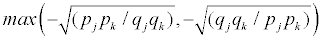and above by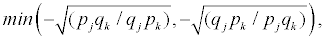where qj=1−pj. Let Φ[x1,x2,ρ] be the cumulative distribution function for a standard bivariate normal random variable with correlation coefficient ρ. Naturally,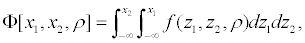where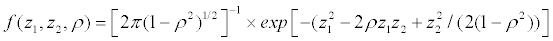. We could generate multivariate normal outcomes (Z’s) whose correlation parameters are obtained by solving the equation

Φ[z(pj), z(pk), ρjk]=δjk(pjqjpkqk)1/2 + pjpk           (1)

for ρjk (j=1,...,d − 1; k=2,...,d) where z(p) denotes the pth quantile of the standard normal distribution. As long as δjk is within the feasible range , the solution is unique. Repeating this numerical integration process d(d−1)/2 times, one can obtain the overall correlation matrix (say Σ) for the d-variate standard normal distribution with mean 0. However, it should be noted that positive semidefiniteness of Σ cannot be guaranteed. To create dichotomous outcomes (Yj) from the generated normal outcomes (Zj), we set Yj=1 if Zj ≥ z(1−pj) and 0 otherwise for j=1,...,d. This produces a vector with the desired properties.

Fleishman polynomials

Fleishman  argued that real-life distributions of variables are typically characterized by their first four moments. He presented a moment-matching procedure that simulates nonnormal distributions often used in Monte Carlo studies. It is based on the polynomial transformation, Y=a+bZ+cZ2+dZ3, where Z follows a standard normal distribution, and Y is standardized (zero mean and unit variance). The distribution of Y depends on the constants a, b, c and d, whose values were tabulated for selected values of skewness (ν1=E[Y3]) and kurtosis (ν2=E[Y4]-3) in the original paper . This procedure of expressing any given variable by the sum of linear combinations of powers of a standard normal variate is capable of covering a wide area in the skewness-elongation plane whose bounds are given by the general expression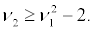Assuming that E[Y]=0, and E[Y2]=1, by utilizing the first 12 moments of the standard normal distribution, the following set of equations can be derived after simple but tedious algebra:

a=−c                              (2)

b2+6bd+2c2+15d2−1=0                    (3)

2c(b2+24bd+105d2+2)−ν1=0                               (4)

24[bd+c2(1+b2+28bd)+d2(12+48bd+141c2+225d2)]−ν20                     (5)

These equations can be solved by the Newton-Raphson method, or any other plausible root-finding or nonlinear optimization routine. More details for the Newton-Raphson algorithm for this particular setting is given by Demirtas and Hedeker , and a computer implementation can be found in Demirtas and Hedeker . Note that the parameters are estimated under the assumption that the mean is 0, and the standard deviation is 1; the resulting data set should be backtransformed to the original scale by multiplying every data point by the standard deviation and adding the observed data mean.

Fleishman’s method has been extended in several ways in the literature. One extension uses the fifth-order polynomials in the spirit of controlling for higher-order moments . The other one is in regard to a multivariate version of the power method  that plays a central role for the remainder of this paper. The procedure for generating multivariate continuous data begins with computation of the constants given in Equations 2-5, for each variable independently. The multivariate case can be formulated in matrix notation as shown below. First, let Z1 and Z2 be variables drawn from standard normal populations; let z′ be the vector of powers 0 through 3, z′=[1, Z, Z2, Z3]; and let w′ be the weight vector that contains the power function weights a, b, c and d, w′=[a, b, c, d]. The nonnormal variable Y is then defined as the product of these two vectors, Y=w′z. Let rY1,Y2 be the correlation between two nonnormal variables Y1 and Y2 that correspond to the normal variables Z1 and Z2, respectively. As the variables are standardized, meaning E(Y1)=E(Y2)=0, rY1Y2=E(Y1Y2)=E(w′1z1z′2w2)= w′1 Rw2, where R is the expected matrix product of z1 and z′2: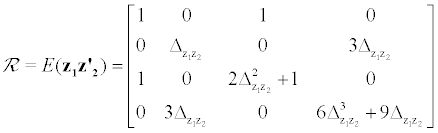where ΔZ1Z2 is the correlation between Z1 and Z2. After algebraic operations, the following relationship between rY1,Y2 and ΔZ1Z2 in terms of polynomial coefficients ensues: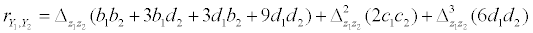(6)

Solving this cubic equation for ΔZ1Z2 gives the intermediate correlation between the two standard normal variables that is required for the desired post-transformation correlation rY1,Y2 . Clearly, correlations for each pair of variables should be assembled into a matrix of intercorrelations that will be used in multivariate normal data generation. For a definitive source and in-depth coverage of Fleishman polynomials .

Correlation and dichotomization

A correlation between two continuous variables is conventionally computed as the common Pearson correlation. A correlation between one continuous and one dichotomous variable is a point-biserial correlation, and a correlation between two dichotomous variables is a phi coefficient (δjk in Equation 1). The point-biserial and phi coefficients are special cases of the Pearson correlation. That is, if we apply the Pearson formula to data involving one continuous and one dichotomous variable, the result will be identical to that obtained using a formula for a point-biserial correlation. Similarly, if we apply the Pearson formula to data involving two dichotomous variables, the result will be identical to that obtained using a formula for a phi coefficient. The point-biserial and phi coefficients are typically used in practice for analyses of relationships involving variables that are true dichotomies . For example, one could use a point-biserial correlation to assess the relationship between sex and cholesterol level, and one could use a phi coefficient to measure the relationship between sex and smoking status (smoker vs. nonsmoker).

Some variables that are measured as dichotomous variables are not true dichotomies. An example would be a situation where the measured variable is dichotomous (obese vs. non-obese), but the underlying variable is continuous (body mass index). Special types of correlations, specifically biserial and tetrachoric correlations, are used to measure relationships involving such artificial dichotomies. Use of these correlations is based on the assumption that underlying a dichotomous measure is a normally distributed continuous variable. For the case of one continuous and one dichotomous variable, a biserial correlation provides an estimate of the relationship between the continuous variable and the other continuous variable underlying the dichotomy. For the case of two dichotomous variables, the tetrachoric correlation (ρjk in Equation 1) describes the relationship between the two continuous variables underlying the measured dichotomies.

Suppose that X and Y follow a bivariate normal distribution with a correlation of ρXY. If X is dichotomized to produce XD, then the resulting correlation between XD and Y can be designated as ρXDY (point-biserial correlation). The effect of dichotomization on ρXY (biserial correlation) is given by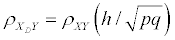(7)

where p and q are the proportions of the population above and below the point of dichotomization, respectively, and h is the ordinate of the normal curve at the same point. The sign of correlation in Equation 7 should not change with dichotomization, so high and low values of X are assigned 1 and 0, respectively. Of note, for the purposes of this work, artificial versus true dichotomies or terminology differences such as “biserial” versus “tetrachoric” are inconsequential.

Obviously, this is all based on normality. However, the specified correlations among nonnormal continuous variables, along with marginal characteristics of nonnormal data are used to compute the corresponding intermediate correlations among normal variables that underlie the nonnormal continuous ones through Equation 6. The way of handling the effect of Fleishman’s  transformation on the correlations between binary-normal and binary-nonnormal continuous pairs is described in Algorithm. Pearson correlations for the normal-normal pairs, phi coefficient for the binary-binary pairs and point-biserial correlation for the binary-normal pairs are obtained within the algorithmic stages, consistent with the dichotomization terminology given in this subsection. After performing calculations given in Equations 1 and 7, one finds an overall Pearson correlation matrix for the underlying multivariate normal realizations before dichotomizing some variables in the system.

After laying the groundwork, I summarize the methodology proposed by Demirtas and Doganay  for generating mixed data in the next section.

Algorithm

Finding the coefficients of powers of normal components of any continuous distribution can be performed by solving a set of nonlinear equations, and employing these coefficients in determining the intermediate correlations among those normal components are explained in Fleishman polynomials. MVN and MVB generation with underlying normal distribution are well-understood, and along with the mathematical connection between point biserial and tetrachoric correlations described in MVN data generation and MVB data generation, one can generate a set of binary and normal variables in a unified manner given marginal proportions and a set of correlations before conducting a transformation from normal to nonnormal variates. More specifically, algorithmic steps are given below. In what follows, B, N and C stand for set of binary, normal and nonnormal continuous variables, respectively.

Let X1,X2, ...,Xj be a set of binary variables with proportion parameters p1,p2...,pj, and let Ym represent the set of continuous variables with known or calculable skewness (ν1m) and kurtosis (ν2m), where m=1,2,...,k. The (j+k)×(j+k) correlation matrix is Σ. Without loss of generality, assume that variables are arranged in a certain order where similar types of variables are grouped together. Then, Σ is comprised of three components: ΣBB, ΣBC, and ΣCC, where B and C correspond to binary and continuous parts, respectively. In this setup, ΣBB is a j×j submatrix and ΣCC is a k×k submatrix of Σ that stand for the correlations between the binary-binary and continuous-continuous combinations, respectively. Similarly, ΣBC represents a j×k submatrix whose elements are the correlations between binary and continuous variables.

Required parameter values (p for binary variables, ν1 and ν2) for continuous variables, and the correlation matrix Σ whose partitions are ΣBC, ΣBB and ΣCC) are either specified or estimated from a real data set that is to be mimicked.

1. Check if Σ is positive semidefinite.

2. Find the upper and lower correlation bounds for the BB part using the information given in MVB data generation.

3. Repeat Step 2 for the BC and CC parts by the approximation method proposed by Demirtas and Hedeker .

4. Make sure all elements of Σ are within the plausible range.

5. Compute tetrachoric correlations for the BB combinations using Equation 1. This has to be done for each and every binary pair, separately.

6. Work with centered and scaled versions of the continuous variables (the mean and standard deviation could be specified via a distribution or come from a real data set). Note that correlations remain unchanged with a linear transformation. Estimate the power coefficients (a,b,c,d) for each of the continuous variable by Equations 2-5 given corresponding ν1 and ν2 values.

7. For each CC combination, using the constants in Step 6, find the intermediate correlation by solving Equation 6.

8. For each BC combination, suppose that two identical standard normal (N) variables, one is the normal component of the continuous variable and the other one is the underlying the binary variable before dichotomization. With this setup,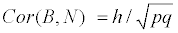using Equation 7, substituting +1 for the biserial correlation (as they are identical before dichotomization).

9. Solve for Cor(C,N) assuming Cor(B,C)=Cor(B,N) * Cor(C,N). It means that the linear association between B and C is assumed to be fully explained by their mutual association with N. In this equation, Cor(B,C) is specified, and Cor(B,N) is found in Step 8.

10. Compute the intermediate correlation between C and N by Equation 6. Notice that for standard normal variables, b=1 and a=c=d=0. So intermediate correlation is the ratio, Cor(C,N)/(b+3d), where b and d are the non-zero coefficients of the nonnormal continuous variable.

11. Construct an overall correlation matrix, Σ* using the results from Steps 5, 7, 8, 9 and 10.

12. Check if Σ* is positive semidefinite. If it is not, find the nearest positive semidefinite correlation matrix.

13. Generate multivariate normal data with a mean vector of (0,...,0)k+j and correlation matrix of Σ*.

14. Obtain binary variables by the thresholds determined by marginal proportions using quantiles of the normal distribution.

15. Obtain continuous variables by the sum of linear combinations of standard normal using the corresponding (a,b,c,d) coefficients.

16. Go back to the original scale for continuous variables by reverse centering and scaling.

There are a few operational issues that need to be addressed. First, two specification violations can occur if the set of parameter values is specified by the user. In Step 1, the correlation matrix Σ should pass the positive semidefiniteness check. In case of failure, the whole process is aborted. Steps 2 and 3 are designed to protect against correlation bound violations. Correlations among variables are typically not free to vary between -1 and 1, with bounds determined by the marginal distributions. The sorting method of Demirtas and Hedeker  can be employed to identify any bound violations that arise from a specification error. If the parameter values are estimated from a complete real data set, positive semidefiniteness condition for Σ must hold and unfeasible correlation range can never ensue. Second, Fleishman polynomials do not cover the entire skewness-elongation plane. Therefore, most but not all not (ν12) specifications are plausible. Third, even when no above-mentioned possible complications exist, the final correlation matrix, Σ*, is not guaranteed to be positive semidefinite. In such cases, I recommend the method of Higham  to proceed with the nearest positive semidefinite correlation matrix. Caveats aside, these days many software packages are capable of performing these algorithmic steps with relative ease from a practical standpoint.

Simulations

The performance of the method has been evaluated in bivariate and multivariate settings with varying underlying distributional shapes via marginal and associational quantities in artificial and real data-based scenarios.

Bivariate case

The bivariate simulations consist of one binary and five different continuous distributions whose densities, key shape characteristics and population values for skewness (ν1) and kurtosis (ν2) are given below.

1. Uniform distribution: f(y|a,b)=(b−a)−1, a ≤ y ≤ b, where a and b are the lower and upper bounds of the support of y. We take (0,1) for (a,b). Here, the density is flat and symmetric around its mean (ν1=0 and ν2=−1.2).

2. Laplace distribution: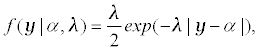where α and λ>0 are the location and inverse scale parameters, respectively. We set α=1, and λ=1. Its shape is symmetric and more peaked than normal (ν1=0 and ν2=3).

3. Normal mixture distribution: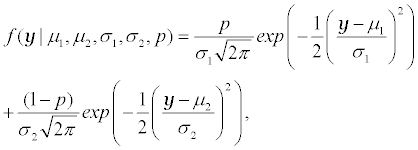where 0<p<1 is the mixing parameter. We set (μ1212,p) to (1,3,1,1,0.5) leading to a symmetric, platykurtic, bimodal density (ν1=0 and ν2=−0.9582).

4. Beta distribution: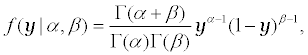where α>0 and β>0 are the shape parameters. We take α=4 and β=2, which makes the shape negatively skewed and less peaked than normal (ν1=−0.4677 and ν2=−0.375).

5. χ2 distribution: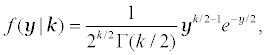y≥0, where k>0 is the degrees of freedom. k is chosen to be 32. The density is positively skewed and leptokurtic (ν1=0.5 and ν2=0.375).

This set of continuous distributions covers flat, unimodal and bimodal symmetric, right-and left-skewed shapes in terms of the third moments (skewness); and leptokurtic and platykurtic shapes in terms of the fourth moments (elongation).

2×2×2=8 combinations were employed where the binary proportion p, the correlation Σ12 and sample size n take the values (0.3,0.5), (−0.3,0.4) and (100,10000), respectively, for each of the five continuous distributions above. The experiment was repeated N=1000 times for each of the 2×2×2×5=40 scenarios. Results for the large sample case were tabulated in Table 1 due to space limitations as the small sample case yielded little or indiscernible deviations. The parameters of interest are three marginal quantities (p,ν12) and one associational quantity (Σ12). The mean estimates that were obtained by averaging the results across N=1000 simulation replicates are reported in Table 1, focusing on the accuracy aspects (the other two simulation studies that we describe in multivariate case and Real data-driven case include a precision measure as well). Very close resemblance between the specified and empirically computed quantities on average throughout all scenarios strongly indicates that the procedure is working properly.

Distribution p Σ12 ν1 ν2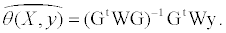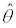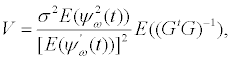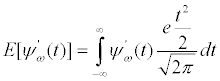Uniform(0, 1) 0.3 -0.3 0 -1.20 0.3000 -0.3013 0.0001 -1.1930
0.3 0.4 0 -1.20 0.3000 0.4009 0.0001 -1.1971
0.5 -0.3 0 -1.20 0.5000 -0.3017 0.0000 -1.1923
0.5 0.4 0 -1.20 0.5000 0.4019 -0.0002 -1.1969
Laplace(1,1) 0.3 -0.3 0 3.0 0.3000 -0.2986 -0.0003 2.9982
0.3 0.4 0 3.0 0.3000 0.3943 0.0007 2.9991
0.5 -0.3 0 3.0 0.5000 -0.2969 -0.0006 3.0130
0.5 0.4 0 3.0 0.5000 0.3988 0.0011 2.9976
Normal mixture 0.3 -0.3 0 -0.9582 0.3000 -0.3015 -0.0002 -0.9581
(1,3,1,1,0.5) 0.3 0.4 0 -0.9582 0.3000 0.4021 -0.0003 -0.9582
0.5 -0.3 0 -0.9582 0.5000 -0.3033 0.0001 -0.9580
0.5 0.4 0 -0.9582 0.5001 0.4008 0.0000 -0.9579
Beta(4,2) 0.3 -0.3 -0.4677 -0.375 0.3000 -0.3009 -0.4673 -0.3756
0.3 0.4 -0.4677 -0.375 0.3000 0.3979 -0.4676 -0.3758
0.5 -0.3 -0.4677 -0.375 0.5000 -0.3019 -0.4681 -0.3751
0.5 0.4 -0.4677 -0.375 0.5000 0.4013 -0.4678 -0.3754
χ2(32) 0.3 -0.3 0.5 0.375 0.3000 -0.2987 0.4996 0.3752
0.3 0.4 0.5 0.375 0.3000 0.4013 0.4998 0.3751
0.5 -0.3 0.5 0.375 0.5000 -0.3000 0.4992 0.3755
0.5 0.4 0.5 0.375 0.5000 0.3999 0.4994 0.3744

Table 1: Specified parameter values for proportion, correlation, skewness and kurtosis, and empirical estimates averaged across N=1000 simulation replicates for five continuous distributions in a bivariate setting with n=10000 data points.

Multivariate case

Following the notation in Algorithm, there are two binary (X1 and X2) with respective proportions p1=0.4 and p2=0.7, and four continuous variables (Y1, Y2, Y3 and Y4) that follow Laplace, Normal mixture, Beta and χ2, respectively, in our simulated multivariate setting. The specified correlation matrix Σ is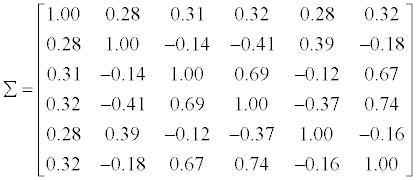After applying the algorithm, the final, overall correlation correlation matrix Σ* of the multivariate normal distribution that plays a central role in obtaining subsequent dichotomization and power transformation to yield the data with desired properties turned out to be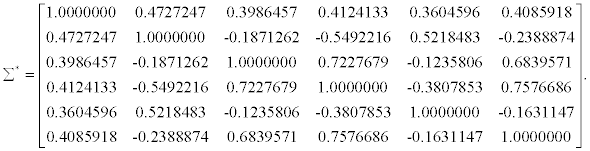Denoting the elements of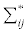where i=1,2,...,6 and j > i, Σ12 (the BB combination) is computed through the relationship between the tetrachoric correlation and phi coefficient given in Equation 1 (Step 5 in the algorithm). The entries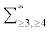(the CC combinations) are calculated by first finding the power coefficients in Step 6 and subsequently finding intermediate correlations via Equation 6 (Step 7). The entries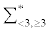(the BC combinations) are computed by Steps 8-10.

The parameters of interest are the odds ratio between X1 and X2 and 15 nonredundant correlation parameters. 1000 simulated data sets were generated with n=1000 rows. The true values (TV), average estimates (AE), raw biases (RB), percentage biases (PB) and 95% coverage rates (CR) across 1000 replicates are shown in Table 2. If the parameter of interest is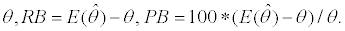AE, RB and PB are accuracy measures, and CR is a hybrid measure of accuracy and precision. Subject to unbiasedness, CRs that are close to the nominal level (95%) suggest that the standard errors are neither too small nor too large; Type 1 and Type II error rates are properly controlled . Of note, we worked with logarithm of odds ratio and Fisher transformed versions of correlations, since they are more likely to follow a normal distribution, which gives more accurate results when we construct confidence intervals. The results tabulated in Table 2 reveal that the average estimates are very similar to the specified values; raw biases are negligibly small and percentage biases are within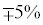(overwhelming majority of them are less than 2% in either direction); and coverage rates are in the neighborhood of the ideal (expected) 95% level.

Parameter TV AE RB PB CR
log(ORX1,X2) 1.40617 1.41273 0.00656 0.47 94.9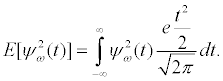0.28 0.27989 -0.00011 -0.04 95.5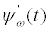0.31 0.30839 -0.00161 -0.52 96.8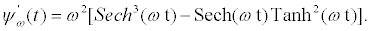0.32 0.32569 0.00569 1.78 95.9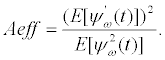0.28 0.27748 -0.00252 -0.90 95.50.32 0.32420 0.00420 1.31 96.4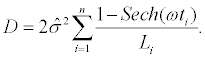-0.14 -0.13793 0.00207 -1.48 95.5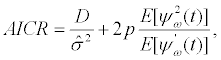-0.41 -0.41520 -0.00520 1.27 94.2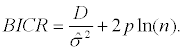0.39 0.40287 0.01287 3.30 92.5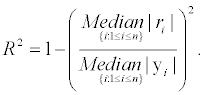-0.18 -0.17933 0.00067 -0.37 94.3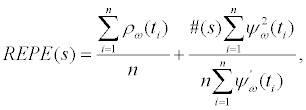0.69 0.68936 -0.00064 -0.09 96.1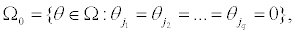-0.12 -0.11858 0.00142 -1.18 95.8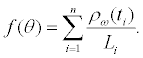0.67 0.66967 -0.00033 -0.05 94.9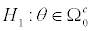-0.37 -0.36874 0.00126 -0.34 94.7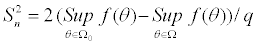0.74 0.73989 -0.00011 -0.02 95.7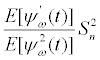-0.16 -0.15885 0.00115 -0.72 94.1

Table 2: Results for one odds ratio and 15 correlation parameters in a multivariate setting with n=1000 rows and N=1000 replications, where X1 and X2 are binary variables with p1=0.4 and p2=0.7; Y1, Y2, Y3 and Y4 follow Laplace (1,1), Normal mixture (1,3,1,1,0.5), Beta (4,2) and χ2 (32) distributions, respectively. T V, AE, RB, P B and C R stand for true value, average estimate, raw bias, percentage bias and coverage rate, respectively.

Real data-driven case

In the data generation process, I simulated one dichotomous variable (DRUG) and four continuous variables (Y0,Y1,Y3,Y6) that correspond to the severity of illness at weeks 0, 1, 3 and 6, respectively, with n=312 subjects as in the original data set.

Specified true values of marginal (proportion of drug patients, skewness and kurtosis of Y s) and associational parameters (correlation matrix of five variables in the data) directly come from the data themselves. In addition to these descriptive measures, model-based quantities (regression coefficients) were also considered.

The regression model is based on well-known linear mixed-effects model . Let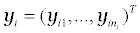denote the responses for subject i. The model is

yi=Xiβ + Zibi + εi,      (8)

where Xi (ni×p) and Zi (ni×q) contain covariates, β contains fixed effects, bi ∼ N(0,ψ) contains random effects, and εi ∼ N(0, σ2Vi). Times of measurement are often incorporated into Xi and Zi, allowing the response trajectories to vary by subject. In the current context, i=1,2,...,312, ni=4, outcome Y denotes illness severity, Xi consists of an intercept, DRUG, WEEK, DRUG * WEEK, Zi includes an intercept and WEEK (random slope model), and Vi is the identity matrix. The parameters of interest are the β coefficients of the terms that appear in the fixed effects regressor matrix. Of note, this may not be the best analysis model, one may build more complex models. However, as long as the same model is used for finding the true parameter values from the original complete data and for analysis after simulating data, using a suboptimal model does not have any impact on the plausibility of data-generation method which is a key theme in this work.

In total, four regression coefficients, four skewness and kurtosis parameters, one proportion and 10 correlation parameters were examined. N=1000 data sets were generated by the characteristics of the data; and the evaluation criteria in Multivariate case were used to assess how unbiased and precise the estimates are. The results are tabulated in Table 3. As before, discrepancies between the average estimates and specified values are almost nonexistent, leading to extremely small biases. Coverage rates are strikingly close to the nominal levels, demonstrating that the magnitude of variability in the system is almost ideal1.

Some logistical and computational details

• All computations were carried out in R .

• Misspecification check in Step 1 was done by is.positive.definite function in R package corpcor .

• All specified correlation terms should be within the feasible range; this has been checked by the method of Demirtas and Hedeker  for each pair of variables (Steps 2 and 3 in the algorithm).

• Tetrachoric correlations in Step 5 were found by tetrachoric function in R package psych .

• Power transformation coefficients in Step 6 were found via the R function given in Demirtas and Hedeker .

• Although it was not needed in this particular work, working with the closest positive semidefinite matrix can be done by nearPD function in R package Matrix , which is an application of Higham .

• Multivariate normal data generation in Step 13 was conducted by rmvnorm function in R package mvtnorm .

• Implementation of linear mixed effects model in the real data application (Real data-driven case) was performed by lmer function in R package lme4 .

#### Discussion

The method is concerned with repeatedly generating synthetic data with specified distributional features or data that on average mimic real data with a mix of binary and continuous variables to assess validity and plausibility of statistical techniques. Parameters that govern the hypothetical process that leads to observed data are either specified by users or preferably estimated from a real data set. The technique relies on well-established multivariate data generation techniques for binary and normal data with added operational utility of power polynomials to preserve marginal characteristics of data as well as the association structure among the variables. There are some points that deserve attention.

• This method does not require specialized tools, it can be implemented by existing software.

• True versus artificial dichotomies and terminological complexities such as phi co- efficient, biserial, point-biserial, tetrachoric correlations are unimportant and just procedural. These are different variants of the Pearson correlation, historically have been used to differentiate correlations among variables of different nature, and computational formula is the same for all.

• As long as initial and final correlation matrices (Σ and Σ*, respectively) are positive semidefinite; correlation bounds among variables are not violated; and symmetry-peakedness (skewnesselongation) behavior for continuous variables is within the region that can be handled by power polynomials, this approach works well (including situations where the binary variables are highly skewed with major proportion of 1s or 0s). If input parameters come from a real complete data set, the initial positive semidefiniteness (Step 1 in the algorithm) and correlation boundary problems (Steps 2 and 3) can never be encountered. However, the final positive semidefiniteness condition (Step 12) may not hold for all given specifications. In this case, one can resort to the technique proposed by Higham , to work with the nearest positive semidefinite correlation matrix.

• The original real data in Real data-driven case have some missing values, but I considered complete cases for the purpose of illustration without regard to missing data issues which are beyond the scope of this manuscript. This is not a limitation, because one can simulate incomplete data set by first generating full data, and then imposing missing values by some nonresponse mechanism [3,13,23-25].

• One appealing feature of this methodology is that simulated variables can be treated as outcomes or predictors in subsequent statistical analyses as the variables are being generated jointly.

• More comprehensive simulation studies with a broader range of parameters could have been investigated, but I believe that these designs adequately accommodate salient marginal parameters such as proportion, skewness and kurtosis, and association parameters such asodds ratio, correlation and regression coefficients.

• As far as continuous part of the data is considered, the method can handle nonnormal features such as skewness, multimodality, boundary at the mode, low or high peakedness.

• This technique is currently designed to accommodate linear associations; modelling nonlinear associations is an important future direction.

• Ideas presented in this paper can be incorporated into the RNG algorithms that involve multivariate ordinal data, proposed by Demirtas  and Demirtas and Yavuz , to produce binaryordinal- continuous combinations.

Given its computational simplicity, generality and flexibility, the method is likely to be a handy addition to practitioners’ toolkit. It is particularly useful for studies that involve longitudinal or clustered designs as well as other situations where multiple binary and continuous variables are collected. When biomedical researchers need to regenerate the original data trends in simulated environments, they could implement this technique in their favorite platform and software with ease.

1Coverage rates for ν1 and ν2 in the real data example were not computed due to heavily nonnormal sampling distribution of these quantities.

#### References

Select your language of interest to view the total content in your interested language

### Article Usage

• Total views: 12614
• [From(publication date):
specialissue-2015 - Sep 19, 2019]
• Breakdown by view type
• HTML page views : 8766

## Post your commentCan't read the image? click here to refresh
###### Peer Reviewed Journals

Make the best use of Scientific Research and information from our 700 + peer reviewed, Open Access Journals

International Conferences 2019-20

Meet Inspiring Speakers and Experts at our 3000+ Global Annual Meetings

Top